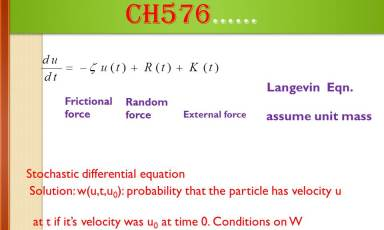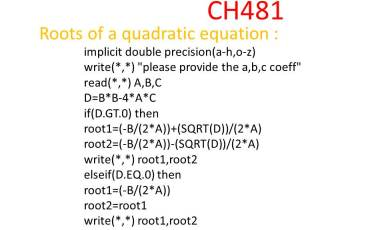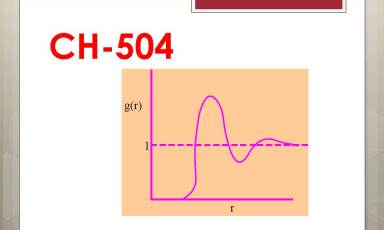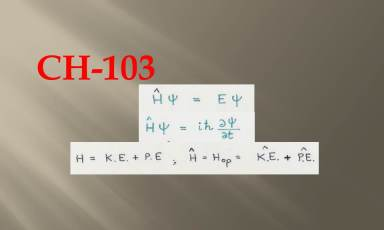Molecular Dynamics Lab### Fundamental equation for non equilibrium statistical mechanics### Fortran code for finding the roots of a quadratic equation### Time independent and time dependent hamiltonian equations

List of courses taught :

• Statistical mechanics in chemistry

• Mathematical methods in chemistry

• Structure and Bonding/ Chemical bond and Molecular Geometry

• Molecular spectroscopy; Quantum Chemistry

• Energetics and kinetics/ Rate Processes

• Chemical and statistical thermodynamics

• Computer methods in chemistry/ Chemistry and computers/ Computational Chemistry

• Physical chemistry and Undergraduate Chemistry laboratories

• Solid state chemistry

• Undergraduate chemistry (Physical Chemistry) - I

• Topics in chemistry:
• (i) FT-NMR methods
• (ii) Dielectric Relaxation
• (iii) Charge transfer processes in chemistry
• (iv) Stochastic Processes

Online Course Materials :

• CH103

• CH504

• CH576

• CH481